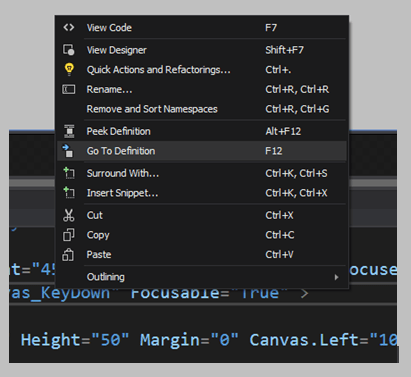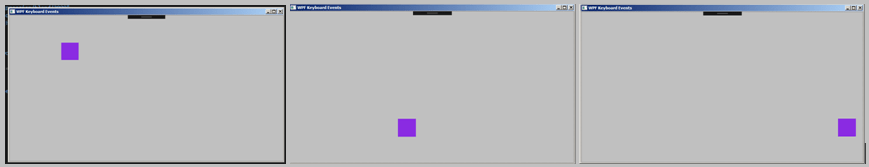# WPF C# Tutorial – Move object with key down events in visual studio

### Start coding the key down events for the programTo add the key down event to the code you can simply right click on the key down part and click on go to definition.

```using System;
using System.Collections.Generic;
using System.Linq;
using System.Text;
using System.Windows;
using System.Windows.Controls;
using System.Windows.Data;
using System.Windows.Documents;
using System.Windows.Input;
using System.Windows.Media;
using System.Windows.Media.Imaging;
using System.Windows.Shapes;

namespace WPF_Keyboard_Events
{
/// <summary>
/// Interaction logic for MainWindow.xaml
/// </summary>
public partial class MainWindow : Window
{
public MainWindow()
{
InitializeComponent();
}

private void Canvas_KeyDown(object sender, KeyEventArgs e)
{

}
}
}```

Above is the key down event added to the app now. We can add our own instructions to move the object across the canvas.

```using System;
using System.Collections.Generic;
using System.Linq;
using System.Text;
using System.Windows;
using System.Windows.Controls;
using System.Windows.Data;
using System.Windows.Documents;
using System.Windows.Input;
using System.Windows.Media;
using System.Windows.Media.Imaging;
using System.Windows.Shapes;

namespace WPF_Keyboard_Events
{
/// <summary>
/// Interaction logic for MainWindow.xaml
/// </summary>
public partial class MainWindow : Window
{
public MainWindow()
{
InitializeComponent();
}

private void Canvas_KeyDown(object sender, KeyEventArgs e)
{
// This is the key down event linked to the canvas
if (e.Key == Key.Down && Canvas.GetTop(rec1) + rec1.Height < 420)
{
// in this if statement we are checking if the down key is pressed
// AND the rec1 objects top plus the height is less than 420 pixels
Canvas.SetTop(rec1, Canvas.GetTop(rec1) + 10);
// if these conditions match then we move the object down 10 pixels
}
else if (e.Key == Key.Up && Canvas.GetTop(rec1) > 0)
{
// in this if statement we are checking if they up key is pressed
// and rec1s top is greater than 10 pixels
Canvas.SetTop(rec1, Canvas.GetTop(rec1) - 10);
// if these conditions are met then we move the object up 10 pixels
}
else if (e.Key == Key.Left && Canvas.GetLeft(rec1) > 0)
{
// in this if statement we are checking if they left key is pressed
// and rec1s left is greater than 0 pixels
Canvas.SetLeft(rec1, Canvas.GetLeft(rec1) - 10);
// if these conditions are met then we move the object left 10 pixels
}
else if (e.Key == Key.Right && Canvas.GetLeft(rec1) + rec1.Width < 790)
{
// in this if statement we are checking if the right key is pressed
// and rec1s right and rec1s width is less than 790 pixels
Canvas.SetLeft(rec1, Canvas.GetLeft(rec1) + 10);
// if these conditions are met then we move the object 10 pixels to the right
}
}
}
}```

Above is the full key down event. Let’s look closer to see how this works. We have 4 different if statements one for each button.

```            if (e.Key == Key.Down && Canvas.GetTop(rec1) + rec1.Height < 420)
{
// in this if statement we are checking if the down key is pressed
// AND the rec1 objects top plus the height is less than 420 pixels
Canvas.SetTop(rec1, Canvas.GetTop(rec1) + 10);
// if these conditions match then we move the object down 10 pixels
}
```

The first if statement is check if the down key is pressed so we are using e.Key == Key.Down and then we are using a double AND sign to say if they key is down AND some other condition. This is a way to use two conditions and make sure the instructions only run if both of them are true. The second condition we are checking is if the rec1’s top plus the rec1 objects height. To access the rectangle from the canvas we are using the Canvas.GetTop(rec1) this command will get the top position of the rectangle and then we add the height of the object to it and we make sure that both of these values are less than 420 pixels. This way the rectangle will stay inside the form.

If the rectangle is still within the form then we can start moving it down. First we used the Canvas.GetTop(rec1) so we now can use another command Canvas.SetTop(rec1) this command will SET a new location for the object, Inside the set top command we will need to give it two values one to identify the object we want to set a new location to and second where do we want to move it. This is why we are using Canvas.SetTop(rec1, Canvas.GetTop(rec1) + 10); Canvas.GetTop(rec1) + 10) is where we are getting the top to move down by 10 pixels. In essence we are capturing the current location of the object and adding 10 pixels to a given direction. Lets take a look at how we can move the object up.

```            else if (e.Key == Key.Up && Canvas.GetTop(rec1) > 0)
{
// in this if statement we are checking if they up key is pressed
// and rec1s top is greater than 10 pixels
Canvas.SetTop(rec1, Canvas.GetTop(rec1) - 10);
// if these conditions are met then we move the object up 10 pixels
}
```

This if statement is similar to the first one in this case you will only few of the syntaxes are different. In this if statement we are checking if the UP key is pressed AND the objects top is greater than 0 meaning its still inside the screen then we can move it up. So if that value drops below 0 we can stop the object from moving thus making sure it stays inside the visible boundary. Let’s move to the left.

```            else if (e.Key == Key.Left && Canvas.GetLeft(rec1) > 0)
{
// in this if statement we are checking if they left key is pressed
// and rec1s left is greater than 0 pixels
Canvas.SetLeft(rec1, Canvas.GetLeft(rec1) - 10);
// if these conditions are met then we move the object left 10 pixels
}
```

So if the left button is pressed and the objects left is greater than 0 then we can keep moving the object left. This way it will stay within the boundary from the LEFT. Now let’s take a look at moving to the RIGHT.

```            else if (e.Key == Key.Right && Canvas.GetLeft(rec1) + rec1.Width < 790)
{
// in this if statement we are checking if the right key is pressed
// and rec1s right and rec1s width is less than 790 pixels
Canvas.SetLeft(rec1, Canvas.GetLeft(rec1) + 10);
// if these conditions are met then we move the object 10 pixels to the right
}
```

In the if statement above is the right button is pressed and the objects right plus the objects width is less than 790 pixels then we keep moving the object right. As you can see there are lots of similarities in the code where we can move the characters in a WPF C# Form.

From all of the examples we have shown to move an object inside the screen also we can ensure that the object stays inside the screen and stops on the edge. Neat.. Lets hit the debug button and see how it works.Click on the start button or you can press F5 button.You have followed this tutorial so far, very well done. Hopefully you enjoyed it now you can make some changes to the program to see how it works and play around with the code. See you on the next one. Moo Out.